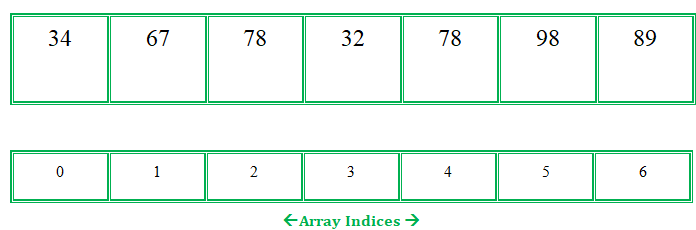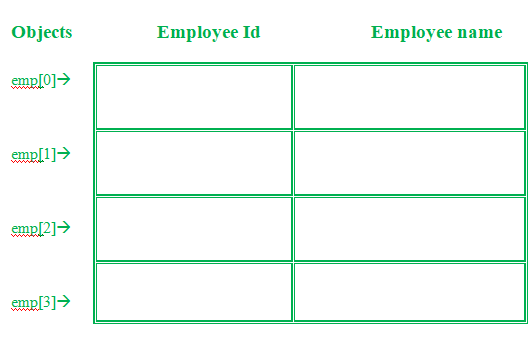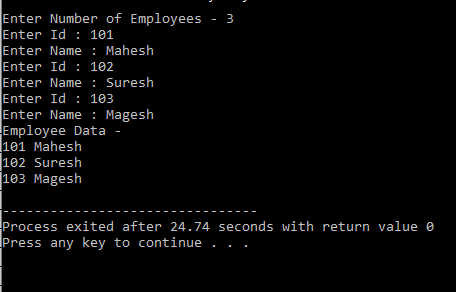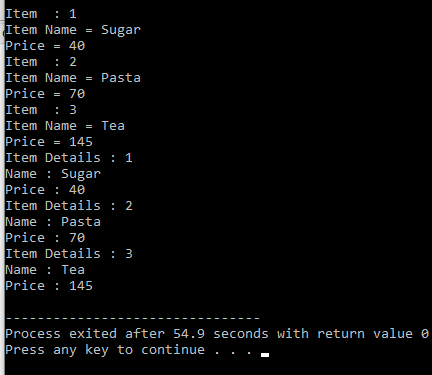Array of Objects in C++ with Examples

• Difficulty Level : Easy
• Last Updated : 17 Nov, 2021

An array in C/C++ or be it in any programming language is a collection of similar data items stored at contiguous memory locations and elements can be accessed randomly using indices of an array.  They can be used to store the collection of primitive data types such as int, float, double, char, etc of any particular type. To add to it, an array in C/C++ can store derived data types such as structures, pointers, etc. Given below is the picture representation of an array.

Example:
Let’s consider an example of taking random integers from the user.Array

Array of Objects

When a class is defined, only the specification for the object is defined; no memory or storage is allocated. To use the data and access functions defined in the class, you need to create objects.

Syntax:

ClassName ObjectName[number of objects];

The Array of Objects stores objects. An array of a class type is also known as an array of objects.

Example#1:
Storing more than one Employee data. Let’s assume there is an array of objects for storing employee data emp.Below is the C++ program for storing data of one Employee:

C++

 // C++ program to implement// the above approach#includeusing namespace std; class Employee{  int id;  char name;  public:  void getdata();//Declaration of function  void putdata();//Declaration of function};void Employee::getdata(){//Defining of function  cout<<"Enter Id : ";  cin>>id;  cout<<"Enter Name : ";  cin>>name;}void Employee::putdata(){//Defining of function  cout<

Let’s understand the above example –

• In the above example, a class named Employee with id and name is being considered.
• The two functions are declared-
• getdata(): Taking user input for id and name.
• putdata(): Showing the data on the console screen.

This program can take the data of only one Employee. What if there is a requirement to add data of more than one Employee. Here comes the answer Array of Objects. An array of objects can be used if there is a need to store data of more than one employee. Below is the C++ program to implement the above approach-

C++

 // C++ program to implement// the above approach#includeusing namespace std; class Employee{  int id;  char name;  public:     // Declaration of function  void getdata();     // Declaration of function  void putdata();}; // Defining the function outside// the classvoid Employee::getdata(){  cout << "Enter Id : ";  cin >> id;  cout << "Enter Name : ";  cin >> name;} // Defining the function outside// the classvoid Employee::putdata(){  cout << id << " ";  cout << name << " ";  cout << endl;} // Driver codeint main(){  // This is an array of objects having  // maximum limit of 30 Employees  Employee emp;  int n, i;  cout << "Enter Number of Employees - ";  cin >> n;     // Accessing the function  for(i = 0; i < n; i++)    emp[i].getdata();     cout << "Employee Data - " << endl;     // Accessing the function  for(i = 0; i < n; i++)    emp[i].putdata();}

Output:Explanation:
In this example, more than one Employee’s details with an Employee id and name can be stored.

• Employee emp – This is an array of objects having a maximum limit of 30 Employees.
• Two for loops are being used-
• First one to take the input from user by calling emp[i].getdata() function.
• Second one to print the data of Employee by calling the function emp[i].putdata() function.

Example#2:

C++

 // C++ program to implement// the above approach#includeusing namespace std;class item{  char name;  int price;  public:  void getitem();  void printitem();};  // Function to get item detailsvoid item::getitem(){  cout << "Item Name = ";  cin >> name;  cout << "Price = ";  cin >> price;   } // Function to print item// detailsvoid item ::printitem(){  cout << "Name : " << name <<          "\n";  cout << "Price : " << price <<          "\n";} const int size = 3; // Driver codeint main(){  item t[size];  for(int i = 0; i < size; i++)  {    cout << "Item  : " <<            (i + 1) << "\n";    t[i].getitem();  }     for(int i = 0; i < size; i++)  {    cout << "Item Details : " <<             (i + 1) << "\n";    t[i].printitem();  }}

Output: